# 值得一看，13个好用到起飞的Python技巧！列表字典Python 是当今广泛使用的编程语言之一，在数据科学、科学计算、Web 开发、游戏开发和构建桌面图形界面等各个领域都有应用。Python 因其在各个领域的实用性、与 Java、C 和 C++ 等其他编程语言相比的生产力以及与英语类似的命令而广受欢迎。

# 列表

## 1. 将两个列表合并到一个字典中

``````keys_list = ['A', 'B', 'C']
values_list = ['blue', 'red', 'bold']

# 有 3 种方法可以将这两个列表转换为字典
# 1.使用Python zip、dict函数
dict_method_1 = dict(zip(keys_list, values_list))

# 2. 使用带有字典推导式的 zip 函数
dict_method_2 = {key:value for key, value in zip(keys_list, values_list)}

# 3.循环使用zip函数
items_tuples = zip(keys_list, values_list)
dict_method_3 = {}
for key, value in items_tuples:
if key in dict_method_3:
pass
else:
dict_method_3[key] = value

print(dict_method_1)
print(dict_method_2)
print(dict_method_3)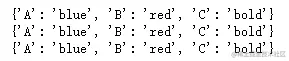## 2.将两个或多个列表合并为一个列表

``````def merge(*args, missing_val = None):
max_length = max([len(lst) for lst in args])
outList = []
for i in range(max_length):
outList.append([args[k][i] if i < len(args[k]) else missing_val for k in range(len(args))])
return outList

merge([1,2,3],['a','b','c'],['h','e','y'],[4,5,6])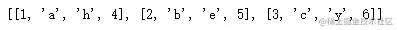## 3. 对字典列表进行排序

``````dicts_lists = [
{
"Name": "James",
"Age": 20,
},
{
"Name": "May",
"Age": 14,
},
{
"Name": "Katy",
"Age": 23,
}
]

# 方法一
dicts_lists.sort(key=lambda item: item.get("Age"))

# 方法二
from operator import itemgetter
f = itemgetter('Name')
dicts_lists.sort(key=f)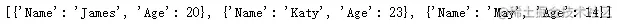## 4. 对字符串列表进行排序

``````my_list = ["blue", "red", "green"]

# 方法一
my_list.sort()
my_list = sorted(my_list, key=len)

# 方法二
import locale
from functools import cmp_to_key
my_list = sorted(my_list, key=cmp_to_key(locale.strcoll))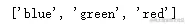## 5. 根据另一个列表对列表进行排序

``````a = ['blue', 'green', 'orange', 'purple', 'yellow']
b = [3, 2, 5, 4, 1]

sortedList =  [val for (_, val) in sorted(zip(b, a), key=lambda x: x)]
print(sortedList)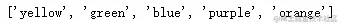## 6. 将列表映射到字典

``````mylist = ['blue', 'orange', 'green']
#Map the list into a dict using the map, zip and dict functions
mapped_dict = dict(zip(itr, map(fn, itr)))

# 字典

## 7. 合并两个或多个字典

``````from collections import defaultdict

def merge_dicts(*dicts):
mdict = defaultdict(list)
for dict in dicts:
for key in dict:
res[key].append(d[key])
return dict(mdict)

## 8. 反转字典

``````my_dict = {
"brand": "Ford",
"model": "Mustang",
"year": 1964
}
# 方法一
my_inverted_dict_1 = dict(map(reversed, my_dict.items()))

# 方法二
from collections import defaultdict
my_inverted_dict_2 = defaultdict(list)
{my_inverted_dict_2[v].append(k) for k, v in my_dict.items()}

print(my_inverted_dict_1)
print(my_inverted_dict_2)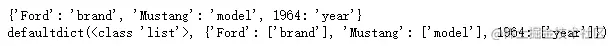# 字符串

## 9. 使用 f 字符串

``````str_val = 'books'
num_val = 15
print(f'{num_val} {str_val}')
print(f'{num_val % 2 = }')
print(f'{str_val!r}')

price_val = 5.18362
print(f'{price_val:.2f}')

from datetime import datetime;
date_val = datetime.utcnow()
print(f'{date_val=:%Y-%m-%d}')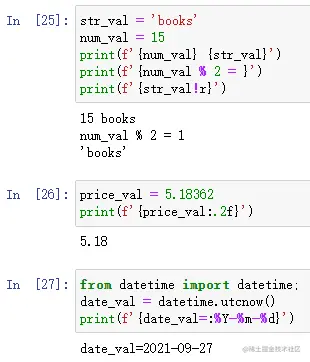## 10. 检查子串

``````addresses = ["123 Elm Street", "531 Oak Street", "678 Maple Street"]
street = "Elm Street"

# 方法一
if address.find(street) >= 0:

# 方法二
if street in address: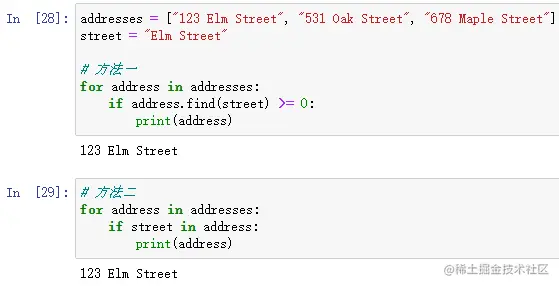## 11. 以字节为单位获取字符串的大小

``````str1 = "hello"
str2 = "😀"

def str_size(s):
return len(s.encode('utf-8'))

print(str_size(str1))
print(str_size(str2))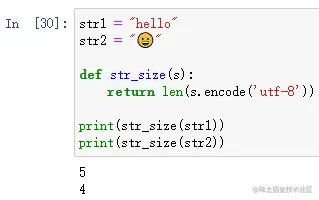# 输入/输出操作

## 12. 检查文件是否存在

``````# 方法一
import os
exists = os.path.isfile('/path/to/file')

# 方法二
from pathlib import Path
config = Path('/path/to/file')
if config.is_file():
pass

## 13.解析电子表格

``````import csv
csv_mapping_list = []
with open("/path/to/data.csv") as my_data:
line_count = 0
for line in csv_reader:
if line_count == 0: